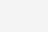# Python数据类型详解

1、字符串
2、布尔类型
3、整数
4、浮点数
5、数字
6、列表
7、元组
8、字典
9、日期

1、字符串
1.1、如何在Python中使用字符串

a、使用单引号(‘)

str=’this is string’;
print str;

b、使用双引号(“)

str=”this is string”;
print str;

c、使用三引号(”’)

str=”’this is string
this is pythod string
this is string”’
print str;

2、布尔类型

bool=False;
print bool;
bool=True;
print bool;

3、整数

int=20;
print int;

4、浮点数

float=2.3;
print float;

5、数字

5.1、删除数字对象引用，例如：

a=1;
b=2;
c=3;
del a;
del b, c;
#print a; #删除a变量后，再调用a变量会报错

5.2、数字类型转换

```int(x [,base]) 将x转换为一个整数
float(x ) 将x转换到一个浮点数
complex(real [,imag]) 创建一个复数
str(x) 将对象x转换为字符串
repr(x) 将对象x转换为表达式字符串
eval(str) 用来计算在字符串中的有效Python表达式,并返回一个对象
tuple(s) 将序列s转换为一个元组
list(s) 将序列s转换为一个列表
chr(x) 将一个整数转换为一个字符
unichr(x) 将一个整数转换为Unicode字符
ord(x) 将一个字符转换为它的整数值
hex(x) 将一个整数转换为一个十六进制字符串
oct(x) 将一个整数转换为一个八进制字符串```

5.3、数学函数

```abs(x)    返回数字的绝对值，如abs(-10) 返回 10
ceil(x)    返回数字的上入整数，如math.ceil(4.1) 返回 5
cmp(x, y) 如果 x < y 返回 -1, 如果 x == y 返回 0, 如果 x > y 返回 1
exp(x)    返回e的x次幂(ex),如math.exp(1) 返回2.718281828459045
fabs(x)    返回数字的绝对值，如math.fabs(-10) 返回10.0
floor(x) 返回数字的下舍整数，如math.floor(4.9)返回 4
log(x)    如math.log(math.e)返回1.0,math.log(100,10)返回2.0
log10(x) 返回以10为基数的x的对数，如math.log10(100)返回 2.0
max(x1, x2,...)    返回给定参数的最大值，参数可以为序列。
min(x1, x2,...)    返回给定参数的最小值，参数可以为序列。
modf(x)    返回x的整数部分与小数部分，两部分的数值符号与x相同，整数部分以浮点型表示。
pow(x, y) x**y 运算后的值。
round(x [,n]) 返回浮点数x的四舍五入值，如给出n值，则代表舍入到小数点后的位数。
sqrt(x)    返回数字x的平方根，数字可以为负数，返回类型为实数，如math.sqrt(4)返回 2+0j```

6、列表
6.1、初始化列表，例如：

list=[‘physics’, ‘chemistry’, 1997, 2000];
nums=[1, 3, 5, 7, 8, 13, 20];

6.2、访问列表中的值，例如：

```'''nums: 1'''
print "nums:", nums
'''nums[2:5]: [5, 7, 8] 从下标为2的元素切割到下标为5的元素，但不包含下标为5的元素'''
print "nums[2:5]:", nums[2:5]
'''nums[1:]: [3, 5, 7, 8, 13, 20] 从下标为1切割到最后一个元素'''
print "nums[1:]:", nums[1:]
'''nums[:-3]: [1, 3, 5, 7] 从最开始的元素一直切割到倒数第3个元素，但不包含倒数第三个元素'''
print "nums[:-3]:", nums[:-3]
'''nums[:]: [1, 3, 5, 7, 8, 13, 20] 返回所有元素'''
print "nums[:]:", nums[:]```

6.3、更新列表，例如：

```nums="ljq";
print nums;```

6.4、删除列表元素

```del nums;
'''nums[:]: [3, 5, 7, 8, 13, 20]'''
print "nums[:]:", nums[:];```

6.5、列表脚本操作符

```print len([1, 2, 3]); #3
print [1, 2, 3] + [4, 5, 6]; #[1, 2, 3, 4, 5, 6]
print ['Hi!'] * 4; #['Hi!', 'Hi!', 'Hi!', 'Hi!']
print 3 in [1, 2, 3] #True
for x in [1, 2, 3]: print x, #1 2 3```

6.6、列表截取

```L=['spam', 'Spam', 'SPAM!'];
print L; #'SPAM!'
print L[-2]; #'Spam'
print L[1:]; #['Spam', 'SPAM!']```

6.7、列表函数&方法

```list.append(obj) 在列表末尾添加新的对象
list.count(obj) 统计某个元素在列表中出现的次数
list.extend(seq) 在列表末尾一次性追加另一个序列中的多个值(用新列表扩展原来的列表)
list.index(obj) 从列表中找出某个值第一个匹配项的索引位置，索引从0开始
list.insert(index, obj) 将对象插入列表
list.pop(obj=list[-1]) 移除列表中的一个元素(默认最后一个元素)，并且返回该元素的值
list.remove(obj) 移除列表中某个值的第一个匹配项
list.reverse() 反向列表中元素，倒转
list.sort([func]) 对原列表进行排序```

7、元组(tuple)

Python的元组与列表类似，不同之处在于元组的元素不能修改；元组使用小括号()，列表使用方括号[]；元组创建很简单，只需要在括号中添加元素，并使用逗号(,)隔开即可，例如：

```tup1 = ('physics', 'chemistry', 1997, 2000);
tup2 = (1, 2, 3, 4, 5 );
tup3 = "a", "b", "c", "d";```

7.1、访问元组

```tup1 = ('physics', 'chemistry', 1997, 2000);
#tup1: physics
print "tup1: ", tup1
#tup1[1:5]: ('chemistry', 1997)
print "tup1[1:5]: ", tup1[1:3]```

7.2、修改元组

tup1 = (12, 34.56);
tup2 = (‘abc’, ‘xyz’);

# 以下修改元组元素操作是非法的。
# tup1 = 100;

# 创建一个新的元组

```tup3 = tup1 + tup2;
print tup3; #(12, 34.56, 'abc', 'xyz')```

7.3、删除元组

```tup = ('physics', 'chemistry', 1997, 2000);
print tup;
del tup;```

7.4、元组运算符7.5、元组索引&截取

```L = ('spam', 'Spam', 'SPAM!');
print L; #'SPAM!'
print L[-2]; #'Spam'
print L[1:]; #['Spam', 'SPAM!']```

7.6、元组内置函数

```cmp(tuple1, tuple2) 比较两个元组元素。
len(tuple) 计算元组元素个数。
max(tuple) 返回元组中元素最大值。
min(tuple) 返回元组中元素最小值。
tuple(seq) 将列表转换为元组。```

8、字典

8.1、字典简介

`dict = {'Alice': '2341', 'Beth': '9102', 'Cecil': '3258'};`

```dict1 = { 'abc': 456 };
dict2 = { 'abc': 123, 98.6: 37 };```

8.2、访问字典里的值

```#!/usr/bin/Python
dict = {'name': 'Zara', 'age': 7, 'class': 'First'};
print "dict['name']: ", dict['name'];
print "dict['age']: ", dict['age'];```

8.3、修改字典

```#!/usr/bin/Python
dict = {'name': 'Zara', 'age': 7, 'class': 'First'};
dict["age"]=27; #修改已有键的值
dict["school"]="wutong"; #增加新的键/值对
print "dict['age']: ", dict['age'];
print "dict['school']: ", dict['school'];```

8.4、删除字典

del dict[‘name’]; # 删除键是’name’的条目
dict.clear(); # 清空词典所有条目
del dict ; # 删除词典

```#!/usr/bin/Python
dict = {'name': 'Zara', 'age': 7, 'class': 'First'};
del dict['name'];
#dict {'age': 7, 'class': 'First'}
print "dict", dict;```

8.5、字典内置函数&方法

```cmp(dict1, dict2) 比较两个字典元素。
len(dict) 计算字典元素个数，即键的总数。
str(dict) 输出字典可打印的字符串表示。
type(variable) 返回输入的变量类型，如果变量是字典就返回字典类型。

9、日期和时间

9.1、获取当前时间，例如：

import time, datetime;

localtime = time.localtime(time.time())
#Local current time : time.struct_time(tm_year=2014, tm_mon=3, tm_mday=21, tm_hour=15, tm_min=13, tm_sec=56, tm_wday=4, tm_yday=80, tm_isdst=0)
print “Local current time :”, localtime9.2、获取格式化的时间

2.1、日期转换为字符串

```首选：print time.strftime('%Y-%m-%d %H:%M:%S');

2.2、字符串转换为日期

```expire_time = "2013-05-21 09:50:35"
d = datetime.datetime.strptime(expire_time,"%Y-%m-%d %H:%M:%S")
print d;```

9.3、获取日期差

```oneday = datetime.timedelta(days=1)
#今天，2014-03-21
today = datetime.date.today()
#昨天，2014-03-20
yesterday = datetime.date.today() - oneday
#明天，2014-03-22
tomorrow = datetime.date.today() + oneday
#获取今天零点的时间，2014-03-21 00:00:00
today_zero_time = datetime.datetime.strftime(today, '%Y-%m-%d %H:%M:%S')

#0:00:00.001000
print datetime.timedelta(milliseconds=1), #1毫秒
#0:00:01
print datetime.timedelta(seconds=1), #1秒
#0:01:00
print datetime.timedelta(minutes=1), #1分钟
#1:00:00
print datetime.timedelta(hours=1), #1小时
#1 day, 0:00:00
print datetime.timedelta(days=1), #1天
#7 days, 0:00:00
print datetime.timedelta(weeks=1)```

9.4、获取时间差

```#1 day, 0:00:00
oneday = datetime.timedelta(days=1)
#今天，2014-03-21 16:07:23.943000
today_time = datetime.datetime.now()
#昨天，2014-03-20 16:07:23.943000
yesterday_time = datetime.datetime.now() - oneday
#明天，2014-03-22 16:07:23.943000
tomorrow_time = datetime.datetime.now() + oneday

print datetime.datetime.strftime(today_time, '%Y-%m-%d %H:%M:%S')
print datetime.datetime.strftime(yesterday_time, '%Y-%m-%d %H:%M:%S')
print datetime.datetime.strftime(tomorrow_time, '%Y-%m-%d %H:%M:%S')```

9.5、获取上个月最后一天

`last_month_last_day = datetime.date(datetime.date.today().year,datetime.date.today().month,1)-datetime.timedelta(1)`

9.6、字符串日期格式化为秒数，返回浮点类型：

```expire_time = "2013-05-21 09:50:35"
d = datetime.datetime.strptime(expire_time,"%Y-%m-%d %H:%M:%S")
time_sec_float = time.mktime(d.timetuple())
print time_sec_float```

9.7、日期格式化为秒数，返回浮点类型：

```d = datetime.date.today()
time_sec_float = time.mktime(d.timetuple())
print time_sec_float```

9.8、秒数转字符串

```time_sec = time.time()
print time.strftime("%Y-%m-%d %H:%M:%S", time.localtime(time_sec))```

http://www.magedu.com/73198.html